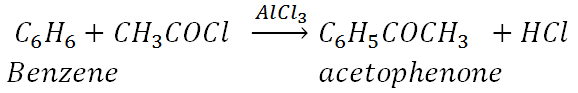MORE IN Applied Chemistry 2
MU First Year Engineering (Semester 2)
Applied Chemistry 2
December 2013
Total marks: --
Total time: --
INSTRUCTIONS
(1) Assume appropriate data and state your reasons
(2) Marks are given to the right of every question
(3) Draw neat diagrams wherever necessary

Answer any five of the following:
1 (a) Define octane number of gasoline. Name any two anti-knock agents.
3 M
1 (b) Why is galvanization of iron articles preferred to tinning?
3 M
1 (c) Give composition properties and uses of Wood's metal.
3 M
1 (d) Explain prevention of waste principle in green chemistry.
3 M
1 (e) Define matrix phase of composite material. Give functions of matrix phase.
3 M
1 (f) State the Characteristics of good paint.
3 M
1 (g) A coal sample was subjected to ultimate analysis. 1.5g of coal on combustion in a bomb calorimeter gave 0.42g of BaSO4. Calculate percentage sulphur in the coal sample.
3 M

2 (a) How do the following factors affect the rate of corrosion?
i. Relative areas of cathodic and anodic parts
ii. Passive character of metal
iii. pH of medium
6 M
2 (b) What is biodiesel? Give trans-esterification reaction in preparation of biodiesel from vegetable oils. What are the advantages of biodiesel?
5 M
2 (c) Calculate the percentage atom economy for the following reaction with respect to acetophenone:-4 M

3 (a) A gaseous fuel has following composition by volume: H2 = 50%, CO = 30%, CH4 = 30%, C2H4 = 5%, N2 = 1%, O2 = 2% and CO2 = 2%. Calculate volume and weight of air required for complete combustion of 1m3 of fuel. (Mol. Wt. of air = 28.949)
6 M
3 (b) Explain conventional and green chemistry route of production of adipic acid. Highlight the green chemistry principle involved.
5 M
3 (c) Discuss differential aeration corrosion with the help of a suitable example.
4 M

4 (a) What are alloy steels? Explain special effects of the following metals on properties of alloy steels:-
i. Chromium
ii. Nickel
iii. Cobalt
iv. Tungsten
6 M
4 (b) What is the principle of cathodic protection method of corrosion control? Explain any one method of corrosion control by cathodic protection.
5 M
4 (c) Write a short note on sandwich panel type layer composites.
4 M

5 (a) What is catalytic cracking? With a schematic diagram, explain any one method of catalytic cracking.
6 M
5 (b) What is powder metallurgy? How are metal powders prepared?
5 M
5 (c) Discuss the influence of any two physical factors on adhesive action.
4 M

6 (a) What is oxidation corrosion? Discuss the role of nature of oxide formed in oxidation corrosion.
5 M
6 (b) A sample of coal has the following composition by weight:- C = 82%, H = 6%, O = 8%, S = 0.5%, N = 3% and Ash = 0.5%. Calculate Gross and net calorific value using Dulong s formula.
5 M
6 (c) What is an alloy? Explain any four purposes of alloying with suitable examples.
5 M

More question papers from Applied Chemistry 2